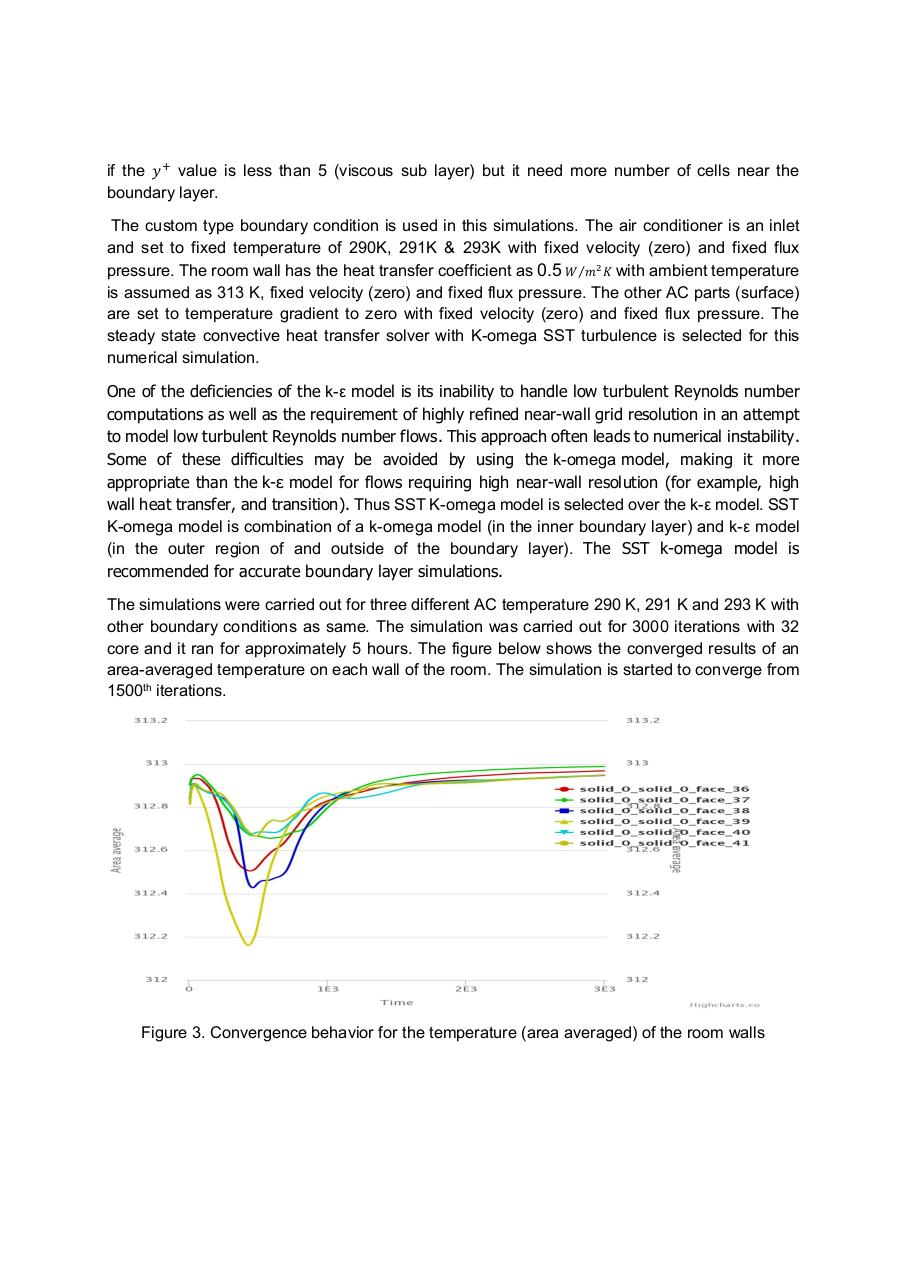# Report performance analysis AC.pdfPage 1 2 3 4 5 6

#### Text preview

if the 𝑦 value is less than 5 (viscous sub layer) but it need more number of cells near the
boundary layer.
The custom type boundary condition is used in this simulations. The air conditioner is an inlet
and set to fixed temperature of 290K, 291K &amp; 293K with fixed velocity (zero) and fixed flux
pressure. The room wall has the heat transfer coefficient as 0.5 𝑊⁄𝑚 𝐾 with ambient temperature
is assumed as 313 K, fixed velocity (zero) and fixed flux pressure. The other AC parts (surface)
are set to temperature gradient to zero with fixed velocity (zero) and fixed flux pressure. The
steady state convective heat transfer solver with K-omega SST turbulence is selected for this
numerical simulation.
One of the deficiencies of the k-ε model is its inability to handle low turbulent Reynolds number
computations as well as the requirement of highly refined near-wall grid resolution in an attempt
to model low turbulent Reynolds number flows. This approach often leads to numerical instability.
Some of these difficulties may be avoided by using the k-omega model, making it more
appropriate than the k-ε model for flows requiring high near-wall resolution (for example, high
wall heat transfer, and transition). Thus SST K-omega model is selected over the k-ε model. SST
K-omega model is combination of a k-omega model (in the inner boundary layer) and k-ε model
(in the outer region of and outside of the boundary layer). The SST k-omega model is
recommended for accurate boundary layer simulations.
The simulations were carried out for three different AC temperature 290 K, 291 K and 293 K with
other boundary conditions as same. The simulation was carried out for 3000 iterations with 32
core and it ran for approximately 5 hours. The figure below shows the converged results of an
area-averaged temperature on each wall of the room. The simulation is started to converge from
1500th iterations.

Figure 3. Convergence behavior for the temperature (area averaged) of the room walls